# Ball rolling down curved ramps

A ball was released from A and it moved along the path as shown in the diagram below.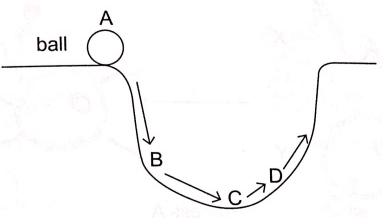Where did the ball possess the greatest
(i) Gravitational potential energy?
(ii) Kinetic energy?

The answer, I thought, was a straightforward (i) A and (ii) C.

Reason is that A is the highest point in the diagram, while C is the lowest point. The PE for C is the lowest, and therefore it would have the highest KE.

Unfortunately, the answer key to the question (ii) was B. It could have been a mistake or a typo, but it set off a lot of debate on why B could be the answer.

I want to thank XXXX, atutor2001, 1girl1boy and Nebbermind for their contribution in the forum, and for the effort in going into much detail and depth trying to justify B or C.

I’ll go through some of these, and why the answer should be C. Note that this is my view and does not represent the views of others. Disclaimer: in the interests of readability, I have paraphrased or simplified some of the arguments put forward. Any misrepresentation is unintentional so please let me know if there is anything I have misquoted.

“B is the end of the steepest part of the section. The ball can’t be rolling faster at C than at B.”

As long as the ball can roll from B to C, it means the ball can pick up speed from B to C. So whatever its speed was at B, it would be faster at C.

“This is a trick question, the answer is B. The original question used to be water falling down a waterfall, landing on an inclined slope. The question setter who chose to use ball is not aware of the significance of the difference between a rolling and a sliding ball.”

There is no trick to this question. If anything, the trick is that B is at the end of the steepest part of the slope. There is no difference between a rolling or sliding ball.  The answer is C, and does not depend on the curvature of the slope, on whether AB is vertical or not, or whether there is a curve at B. A ball was most likely chosen because it is least affected by friction.

“The ball is shown only making it halfway up the pipe. So B must have been fastest.”

First, we cannot assume the ball only made it halfway up the pipe. This was not stated in the question.

Second, we can only conclude that the fastest point would be somewhere before C. Exactly how far before would not be possible to determine without knowing more parameters, such as the curvature of the slope and the properties of the materials. We do not know if that point will be nearer to B or nearer to C.

Third, the location of B could be arbitrarily defined and we cannot always choose the first point before C. For example, if B was quite a fair distance from C, would we have to guess whether B is close enough to the point of maximum KE?

Since the question is testing on PE and KE, the answer C is most appropriate.

“The vertical velocity is lost when the ball rolls to C since C has no vertical velocity component.”

The vertical velocity isn’t lost. It is still kinetic energy and it is still conserved, whatever the angle of the slope. Galileo once proved that the speed gained in rolling down a ramp of given height didn’t depend on the slope. He concludes that for a ball rolling down a ramp, the speed at various heights is the same as the speed the ball would have attained (much more quickly!) by just falling vertically from its starting point to that height. (http://galileoandeinstein.physics.virginia.edu/lectures/gal_accn96.htm)

“Friction is the force that is changing the direction of the ball. Since the ball changes direction, friction must be large, therefore B must have the highest KE.”

It is the normal force (the force that acts perpendicular to the surface) that changes the direction of the ball. The normal force, by definition, acts perpendicular to the surface, while the direction of motion is parallel to the surface. This normal force gives rise to an acceleration that changes the direction of motion but does not add to or subtracts from its velocity. (http://faculty.bucks.edu/taylors/Math242/VelocAndAccel.pdf)

“The ball is hitting the slope at an incline, so energy must have been lost since the velocity after hitting the incline must be v sin θ.”

The ball is running down a curved slope. There is no collision.

Even if there was a collision, the velocity after hitting the incline isn’t v sin θ. To determine the final velocity, we will need to know more about the parameters of the collision.

“The ball slows down because the energy is used to rotate the ball.”

Indeed, the ball slows down if the energy is transferred into rotational energy of the ball. However, rotational energy is still kinetic energy, so the sum of the KE remains the same. (http://en.wikipedia.org/wiki/Rotational_energy)

“There will be impact if the ball isn’t rolling. The impact will cause a large loss in energy and the ball will slow down.”

The ball is rolling down a curved slope. If it isn’t rolling, when it first touches the curved slope, friction is low, because the surface is close to vertical. This friction can cause the ball to roll. If the ball doesn’t roll fast enough, it can slide. Since the slope is curved, there is no sudden deceleration.

Also, if there is impact at B, we do not know if B represents the moment before impact, or after impact. If B is the moment after impact, then C is still the most likely answer.

“The KE is not always highest at the lowest point of the path. If the slope is steep at the start and it becomes gentle towards the end, then friction loss is great and most of the KE gain from the steep fall may be lost”

As long as there is downward sloping slope, there is acceleration and there will be a KE gain down the slope. The difficulty with the above statement is “how steep is steep, and how gentle is gentle?” The student cannot be expected to guess whether AB is considered steep and BC is considered gentle.

Summary

The answer should be C because:

1. It is the simplest explanation, and corresponds with the PSLE syllabus. The explanation is that the PE is at the minimum at the lowest point. All of the PE must have been converted to KE (in the absence of friction) so KE must be highest at C.

2. The speed gained in rolling down a ramp of given height doesn’t depend on the slope. (http://galileoandeinstein.physics.virginia.edu/lectures/gal_accn96.htm) This can be proven with calculus. This means that the final KE depends only on the height on which the object falls from.

3. The ball is likely to accelerate from B to C, so C is still the most likely answer.

4. We do not need to guess if B is closer to the point of maximum KE than C is.

5. We do not need to guess if B is the moment before, or after an impact (if any).

6. We do not need to guess if the slope AB is considered steep and BC is considered gentle.

7. We do not need to assume where the ball stops on its first pass.

Other illustrations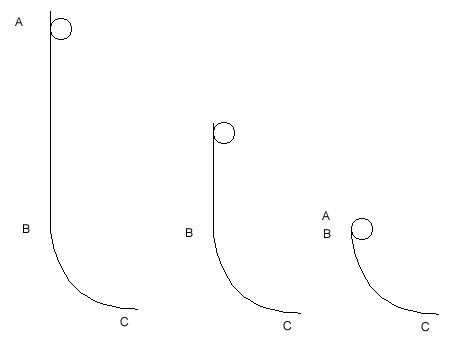KE at C is highest in the leftmost figure.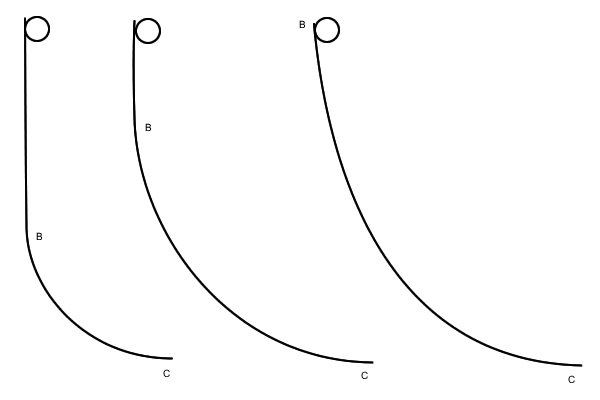KE at C is the same in all three, since the balls are released from the same height.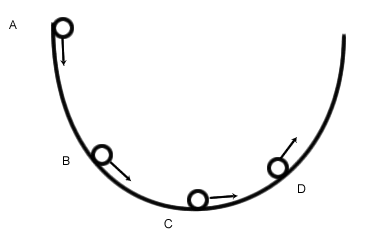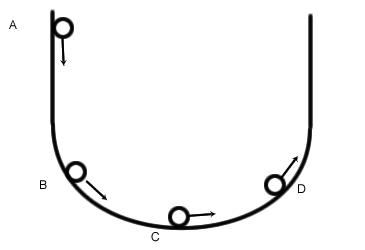In these two diagrams, the answers are both C.

References:

Roller coaster physics – http://vip.vast.org/BOOK/PDFFILES/PART1A.PDF
Galileo on ramps – http://galileoandeinstein.physics.virginia.edu/lectures/gal_accn96.htm
Perpendicular forces changing direction of motion – faculty.bucks.edu/taylors/Math242/VelocAndAccel.pdf
Textbook chapter on kinetic energy and work – http://www.wiley.com/college/sc/cummings/PUBLISHED_09_p226-258.pdf